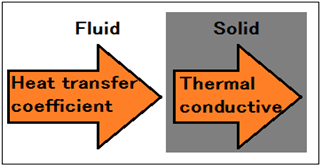MobilePC

PC site display

# 4-2.Drying speed and wind speed of the hot airTemperature and Humidity of the hot air is called the steady-state drying conditions drying constant.

### 4-2-1.If wind velocity of a hot wind is enlarged, a coefficient of heat transfer will also become large.

Heat transfer coefficient of the dry conditions is proportional to the wind speed of √2.

h ∝ √2u

Therefore, the constant drying rate is also proportional to √2.

Rc ∝ √2u

u  : Fluid speed (m/s)
h  : Heat transfer coefficient
Rc : Constant drying rate
C  : Specific heat(J/kg・K)
ρ : Density(kg/m3)
μ : Viscosity (Pa・s)
κ : Thermal conductivity(W/m・K)

### 4-2-2.The air pressure and the amount of air flow.

Relationship of flow and pressure of the air flowing through the pipe is

Q=C×A×V   These relation will be such the expression.

Q : amount of air flow
C : runoff coefficient
A : flow area
V : flow speed
P : pressure
ρ: Density

V=(2×Ｐ÷ρ)^0.5 Than the Bernoulli’s law.

Ｑ＝Ｃ×Ａ×(2×Ｐ÷ρ)^0.5